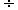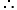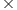Chemistry
Easy

Question

# Find the weight of water present in 1.61 g of Na2SO4 .10H2O?

## 0.8gm0.9gm10gm0.7gmHint:

## The correct answer is: 0.9gm

### Molecular mass of Na2SO4.10H2O = 2(23) + 32 + 4(16) + 10(18) = 322 g, Where atomic mass of Na, S,O are 23g, 32g, 16g respectively and molecular mass of H2O is 18g.For 1 mole of Na2SO4.10H2O, 180g of water is present.Number of moles in 1.61g = 1.61322 = 0.005.The weight of water present in 1.61 g of Na2SO4 .10H2O = (0.005180) g = 0.9 gm#### With Turito Foundation.#### Get an Expert Advice From Turito.